# Optical Lenses Principles and Applications

#### Refraction of Light Rays in Different Situations

We first need to understand refraction of light rays in order to examine how convex and concave lenses work.
We will study the angle of deviation of the refracted ray from the normal to the interface. We consider a light ray incident on a medium with refractive index n1 into a medium of refractive index n2. We will consider 3 different cases where n1 < n2, n1 > n2 and the special case where the angle of incidence is equal to 0.
case 1) n1 < n2
According to Snell's law we have
n1 sin(i) = n2 sin (t) which may be written (n1 / n2) sin(i) = sin (t)
n1 < n2 can be written as n1 / n2 < 1
Multiply all terms of the above inequality by sin(i) to obtain
(n1 / n2) sin(i) < sin(i)
Substitute (n1 / n2) sin(i) by sin(t) to obtain
sin(t) < sin(i)
Which gives: t < i
Meaning that angle t is smaller than angle i or the refracted ray is bent towards the normal to the interface. So in going from a material with lower optical density to a material with higher optical density, the ray is refracted towards the normal to the interface. (See diagram below)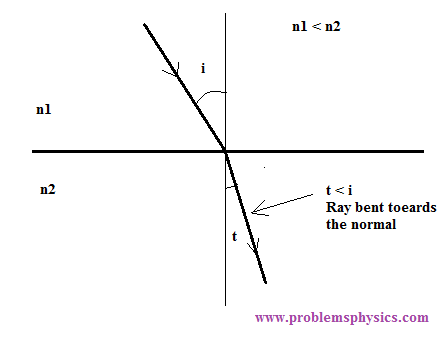case 2) n1 > n2
According to Snell's law we have
(n1 / n2) sin(i) = sin (t) (see above)
n1 > n2 can be written as n1 / n2 > 1
Multiply all terms of the above inequality by sin(i) to obtain
(n1 / n2) sin(i) > sin(i)
Substitute (n1 / n2) sin(i) by sin(t) to obtain
sin(t) > sin(i)
Which gives: t > i
Meaning that angle t is larger than angle i or the refracted ray is bent away from the normal to the interface. When passing from a more to a less optically dense materials, the ray is refracted away from the normal.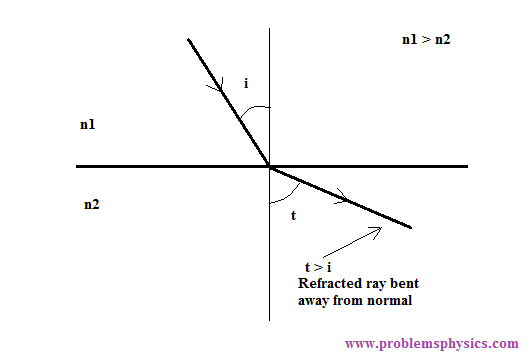case 3) angle of incidence is equal to 0
The ray is transmitted into medium 2 with an angle equal to zero as shown below.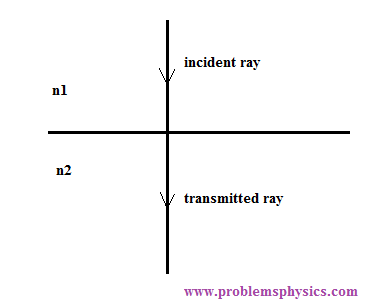Example 1
In the diagram below, a ray of light is incident in medium (1) and travels through different mediums. The path of the ray indicates that the angle of refraction increases form medium (1) to medium (5).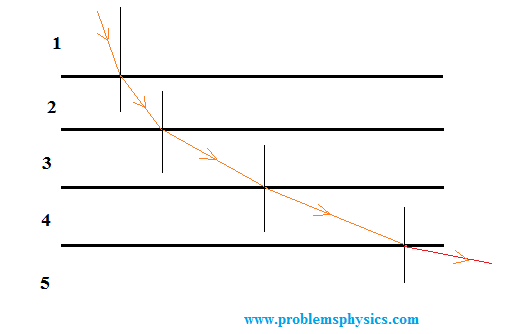a) Which medium has the lowest refractive index?
b) Which medium has the lowest speed of light?
Solution
a)
First the angle of refraction in medium (2) is larger than the angle of incidence in medium (1). Hence the refractive index of medium (1) is larger than the refractive index of medium (2). As we go from medium (2) to medium (5) the angle of refraction increases. Hence the refractive index decreases from medium (1) to medium (5). Medium (5) has the lowest refractive index.
b)
The refractive index n of a medium is defined as the ratio of the speed of light in vacuum c (constant) and the speed of light v of the medium.
n = c / v
v = c / n
According to part a) above, medium (1) has the highest refractive index and therefore medium (1) has the lowest speed of light according the formula v = c / n above.

#### Converging Lenses Principles

In the figure below, a horizontal ray of light is incident in air onto the side AB of triangular piece of glass. The refractive index of the glass (more optically dense) is larger than 1 (refractive index of air, less optically dense), the ray is therefore refracted towards (angle r) the normal to AB at point M. A second refraction at point N where the ray is going from glass (more optically dense) to air (less optically dense) will refract the ray away from the normal to BC at N with an overall deviation of the ray from the original direction (horizontal) downward.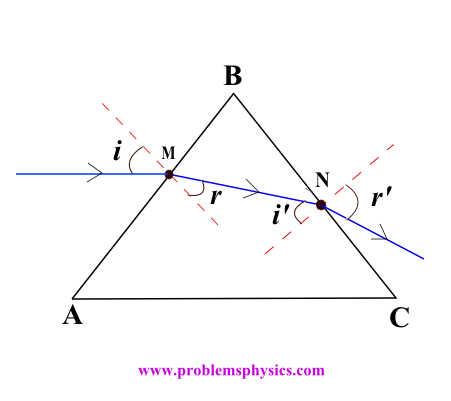Based on the above, it is possible to design a piece of glass shaped in such a way that it refracts many incoming rays parallel to its axis that would converge towards a common point called focal point (focus) as shown below and this is called a converging convex lens. (shown below)#### Diverging Lenses Principles

In the figure below, the incoming incident ray on AB is refracted towards the normal (from less to more optically dense mediums) and away from the horizontal direction. A second refraction at N will refract the ray away from the normal (from more to less optically dense mediums) at N and hence further away and up from the (horizontal) direction of the original ray.Based on the above, it is possible to design a piece of glass shaped in such a way that it refracts many incoming rays parallel (to its axis) that would diverge away from the horizontal direction as shown below and this called divergent concave lens (shown below).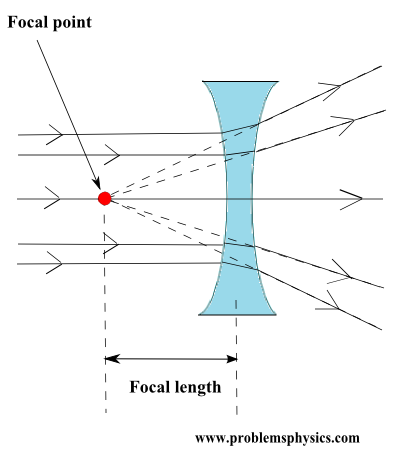#### Optical Lenses Applications

Optical lenses are found in or used in

• our eyes
• astronomical telescopes
• binoculars
• magnifying glasses
• microscopes
• eye glasses and contact lenses